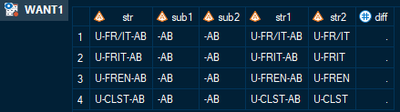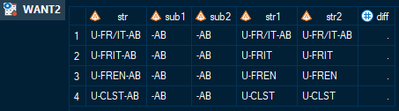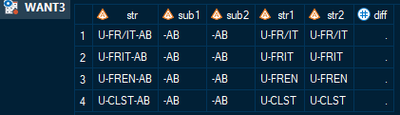## Problem with SCAN and TRANSTRN functions related to variable lengths

Hi SAS people,

I'm wondering why when I use the SCAN function to extract a substring into a new variable which I then use as the second argument (target) of the TRANSTRN function in a subsequent line of code, the resulting variable created from the TRANSTRN function does not remove/replace the target substring whereas the exact same substring just manually created as a new variable (i.e., without using the SCAN function) does result in the target substring having been removed when it is specified in that second argument of the TRANSTRN function. Unless I specify the exact length of the targeted substring beforehand...

I.e., given this data set

```data have;
infile datalines truncover;
input str \$10.;
datalines;
U-FR/IT-AB
U-FRIT-AB
U-FREN-AB
U-CLST-AB
;```

what I would like to see in a resulting data set is the "-AB" at the end of each value for str removed, resulting in "U-FR/IT", "U-FRIT", "U-FREN", and "U-CLST", respectively.

Here are three versions of the code I am using to accomplish this, each with the resulting table. Notice I also create another variable (sub2) with the target substring ("-AB") manually, which I then show is in fact the same exact value by comparing the two in a variable called "diff":

```data want1 (drop=x); set have;
x=compbl(scan(str,3,"-"));
sub1=compbl(cats("-",compbl(x)));
sub2="-AB";

str1=transtrn(str,sub1,trimn(""));
str2=transtrn(str,sub2,trimn(""));

if sub1 ne sub2 then diff=1;
run;``````data want2 (drop=x); set have;
length x \$4; format x \$4.;
length sub1 \$4; format sub1 \$4.;
length sub2 \$4; format sub2 \$4.;
x=compbl(scan(str,3,"-"));
sub1=compbl(cats("-",compbl(x)));
sub2="-AB";

str1=transtrn(str,sub1,trimn(""));
str2=transtrn(str,sub2,trimn(""));

if sub1 ne sub2 then diff=1;
run;``````data want3 (drop=x); set have;
length x \$3; format x \$3.;
length sub1 \$3; format sub1 \$3.;
length sub2 \$3; format sub2 \$3.;
x=compbl(scan(str,3,"-"));
sub1=compbl(cats("-",compbl(x)));
sub2="-AB";

str1=transtrn(str,sub1,trimn(""));
str2=transtrn(str,sub2,trimn(""));

if sub1 ne sub2 then diff=1;
run;```So my question is: do I seriously need to specify lengths (and maybe formats) of the variable resulting from the SCAN function if I want to use it properly in a subsequent TRANSTRN function? What if the lengths are variable? Do I need to extract the length of each result and then create new variables for each length? Also, why the heck is the length of \$4 in the WANT2 dataset working for the three values of str without a forward slash, but not for the one with the forward slash in row 1?

Any insight into this strange issue (strange to me) would be much appreciated!

1 ACCEPTED SOLUTION

Accepted Solutions

## Re: Problem with SCAN and TRANSTRN functions related to variable lengths

It is a very good idea to set the lengths of character variables because otherwise the first use of the variable will set the length.

Depending on that use your variable could be 1 character, 10 or 200.

Consider this example:

```data have;
infile datalines truncover;
input str \$10.;
x=cats(str,'abc');
datalines;
U-FR/IT-A
U-FRIT-AB
U-FREN-AB
U-CLST-AB
;```

Str is 10 characters, the CATS function adds three characters. So how long is X? 200. From the documentation of the CATS function:

In a DATA step, if the CATS function returns a value to a variable that has not previously been assigned a length, then that variable is given a length of 200 bytes.

From the documentation of the scan function:

• In a DATA step, if the SCAN function returns a value to a variable that has not yet been given a length, that variable is given the length of the first argument.

So your code when you do not assign lengths to the result of the scan function is the length of the Str variable.

If your headache revolves around defining lengths then do not create additional variables, nest the function calls:

```data have;
infile datalines truncover;
input str \$10.;

str1=transtrn(str,strip(cats('-',scan(str,'3','-'))),trimn(""));
datalines;
U-FR/IT-A
U-FRIT-AB
U-FREN-AB
U-CLST-AB
;```

Unless you really have a different format that you want don't bother to assign formats to character variables. The default format is \$w. where w is the length.

4 REPLIES 4

## Re: Problem with SCAN and TRANSTRN functions related to variable lengths

It is a very good idea to set the lengths of character variables because otherwise the first use of the variable will set the length.

Depending on that use your variable could be 1 character, 10 or 200.

Consider this example:

```data have;
infile datalines truncover;
input str \$10.;
x=cats(str,'abc');
datalines;
U-FR/IT-A
U-FRIT-AB
U-FREN-AB
U-CLST-AB
;```

Str is 10 characters, the CATS function adds three characters. So how long is X? 200. From the documentation of the CATS function:

In a DATA step, if the CATS function returns a value to a variable that has not previously been assigned a length, then that variable is given a length of 200 bytes.

From the documentation of the scan function:

• In a DATA step, if the SCAN function returns a value to a variable that has not yet been given a length, that variable is given the length of the first argument.

So your code when you do not assign lengths to the result of the scan function is the length of the Str variable.

If your headache revolves around defining lengths then do not create additional variables, nest the function calls:

```data have;
infile datalines truncover;
input str \$10.;

str1=transtrn(str,strip(cats('-',scan(str,'3','-'))),trimn(""));
datalines;
U-FR/IT-A
U-FRIT-AB
U-FREN-AB
U-CLST-AB
;```

Unless you really have a different format that you want don't bother to assign formats to character variables. The default format is \$w. where w is the length.

## Re: Problem with SCAN and TRANSTRN functions related to variable lengths

Thanks for this! I'm not sure why I didn't just think to nest the function calls.

I guess I wasn't sure why the length mattered when it came to the actual values, which, as the diff variable showed, were identical to the one I had created. When, generally, is the length of a character variable important? What really threw me was why in the WANT2 data, the ones without the forward slash worked but not the one with the forward slash in the original string--why should that have made a difference if it wasn't even part of the substring extracted from the SCAN function?

## Re: Problem with SCAN and TRANSTRN functions related to variable lengths

@robeldritch wrote:

Thanks for this! I'm not sure why I didn't just think to nest the function calls.

I guess I wasn't sure why the length mattered when it came to the actual values, which, as the diff variable showed, were identical to the one I had created. When, generally, is the length of a character variable important? What really threw me was why in the WANT2 data, the ones without the forward slash worked but not the one with the forward slash in the original string--why should that have made a difference if it wasn't even part of the substring extracted from the SCAN function?

I think you'll find it was because  the Sub1 and Sub2 still have the trailing blanks. When a value is used with strings generally the whole length is considered to some extent. The other values of STR than the first had a trailing space that matches in the Sub1 and Sub2 but not for the first.

COMPBL does not permanently remove the position in the defined length, just for the duration of that function use.

So if you strip off the trailing blanks in the Transtrn call at the time the variable is used then that trailing blank is completely ignored.

```data want2 (drop=x); set have;
length x \$4; format x \$4.;
length sub1 \$4; format sub1 \$4.;
length sub2 \$4; format sub2 \$4.;
x=compbl(scan(str,3,"-"));
sub1=compbl(cats("-",compbl(x)));
sub2="-AB";

str1=transtrn(str,strip(sub1),trimn(""));
str2=transtrn(str,sub2,trimn(""));

if sub1 ne sub2 then diff=1;
run;```

I have to remember this about once every two or three months when one of my variables that previously didn't have a varying length starts misbehaving.

## Re: Problem with SCAN and TRANSTRN functions related to variable lengths

Ok--that makes sense. Thanks! (I apologize for the delay--didn't see this notification until now).

Discussion stats
• 4 replies
• 263 views
• 2 likes
• 2 in conversation